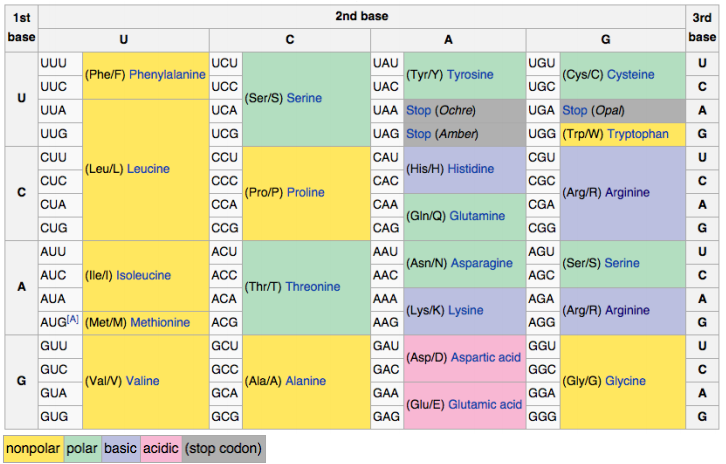# Problem: Given the DNA sequences below of coding strands, identify and underline the mutation in each. What effect did each DNA mutation have on the final protein products. What type of mutation occurred (synonymous, non-synonymous, nonsense, nonstop)? Explain. Original DNA Sequence5’ – A T G T T T C T G G T C A A T T G C G C A G G T C C A A C C T A G – 3’Mutated Sequences:A. 5’ – A T G T T T T T G G T C A A T T G C G C A G G T C C A A C C T A G – 3’B. 5’ – A T G T T T G T G G T C A A T T G C G C A G G T C C A A C C T A G – 3’C. 5’ – A T G T T T C T G G T C A A T T G C G C A G G T C C A A C C T A A – 3’D. 5’ – A T G C T T T C T G G T C A A T T G C G C A G G T C C A A C C T A G – 3’E. 5’ – A T G T T T C T G G T C A A T T A G C G C A G G T C C A A C C T A G – 3’F. Which of the above mutations do you think would be more deleterious to the cell/organism? Explain your reasoning.

###### Problem Details

Given the DNA sequences below of coding strands, identify and underline the mutation in each. What effect did each DNA mutation have on the final protein products. What type of mutation occurred (synonymous, non-synonymous, nonsense, nonstop)? Explain. Original DNA Sequence

5’ – A T G T T T C T G G T C A A T T G C G C A G G T C C A A C C T A G – 3’

Mutated Sequences:

A. 5’ – A T G T T T T T G G T C A A T T G C G C A G G T C C A A C C T A G – 3’

B. 5’ – A T G T T T G T G G T C A A T T G C G C A G G T C C A A C C T A G – 3’

C. 5’ – A T G T T T C T G G T C A A T T G C G C A G G T C C A A C C T A A – 3’

D. 5’ – A T G C T T T C T G G T C A A T T G C G C A G G T C C A A C C T A G – 3’

E. 5’ – A T G T T T C T G G T C A A T T A G C G C A G G T C C A A C C T A G – 3’

F. Which of the above mutations do you think would be more deleterious to the cell/organism? Explain your reasoning.